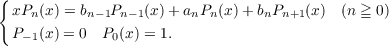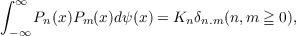#### Vol. 49, No. 2, 1973

 Download this articleFor screen For printingRecent Issues Vol. 325: 1 Vol. 324: 1  2 Vol. 323: 1  2 Vol. 322: 1  2 Vol. 321: 1  2 Vol. 320: 1  2 Vol. 319: 1  2 Vol. 318: 1  2Online Archive Volume: Issue:The Journal Subscriptions Editorial Board Officers Contacts Submission Guidelines Submission Form Policies for Authors ISSN: 1945-5844 (e-only) ISSN: 0030-8730 (print) Special Issues Author Index To Appear Other MSP Journals
On finding the distribution function for an orthogonal polynomial set

### Wm. R. Allaway

Vol. 49 (1973), No. 2, 305–310
##### Abstract

Let {an}n=0 and {bn}n=0 be real sequences with bn > 0 and {bn}n=0 bounded. Let {Pn(x)}n=0 be a sequence of polynomials satisfying the recurrence formula(1.1)

Then there is a substantially unique distribution function ψ(t) with respect to which the Pn(x) are orthogonal. That is,where Kn0 and δn,m is the kronecker delta. This paper gives a method of constructing ψ(x) for the case limn→∞b2n = 0, limn→∞b2n+1 = b < , the set of limit points of {αn}n=1 equals {−α,α} and limn→∞{a2n + a2n+1} = 0. The same method can be used in the case limn→∞bn = 0 and the set of limit points of {an}n=0 is bounded and finite in number.

Primary: 42A52
##### Milestones